# AP Board 7th Class Maths Solutions Chapter 2 Fractions, Decimals and Rational Numbers Ex 2.3

SCERT AP 7th Class Maths Solutions Pdf Chapter 2 Fractions, Decimals and Rational Numbers Ex 2.3 Textbook Exercise Questions and Answers.

## AP State Syllabus 7th Class Maths Solutions 2nd Lesson Fractions, Decimals and Rational Numbers Exercise 2.3

Question 1.
Fill ¡n the blanks in the table. One is done for you.

 Division Quotient 1. 362.21 ÷ 10 36.221 2. 5636.1 ÷ 100 56.361 3. 374.9 ÷ 1000 0.3749 4. 2016.4 ÷ 1000 2.0164 5. 123.0 ÷100 1.23 6. 1300.7 ÷ 1000 1.3007 7. 590.01 ÷ 10 59.001

Question 2.
Solve the following,
(i) 5.51 ÷ 2
5.51 ÷ 2
= $$\frac{551}{100} \div \frac{2}{1}$$
= $$\frac{551}{100} \times \frac{1}{2}$$
= $$\frac{551 \times 1}{100 \times 2}$$
= $$\frac{551}{200}$$
∴ 5.51 ÷ 2 = 2.755

(ii) 38.4 ÷ 3
38.4 ÷ 3
= $$\frac{384}{10} \div \frac{3}{1}$$
= $$\frac{384}{10} \times \frac{1}{3}$$
= $$\frac{384 \times 1}{10 \times 3}$$
= $$\frac{384}{30}$$
∴ 38.4 ÷ 3 = 12.8

(iii) 57.39 ÷ 6
57.39 ÷ 6
= $$\frac{5739}{100} \div \frac{6}{1}$$
= $$\frac{5739}{100} \times \frac{1}{6}$$
= $$\frac{5739 \times 1}{100 \times 6}$$
= $$\frac{5739}{600}$$
∴ 57.39 ÷ 6 = 9.565(iv) 562.1 ÷ 11
562.1 ÷ 11
= $$\frac{5621}{10} \div \frac{11}{1}$$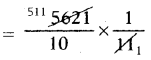= $$\frac{511}{10}$$
∴ 562.1 ÷ 11 = 51.1

(v) 0.7005 ÷ 5
0.7005 ÷ 5
= $$\frac{7005}{10000} \div \frac{5}{1}$$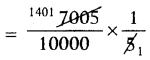= $$\frac{1401}{10000}$$
∴ 0.7005 ÷ 5 = 0.1401

(vi) 9.99 ÷ 3
9.99 ÷ 3
= $$\frac{999}{100} \div \frac{3}{1}$$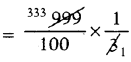= $$\frac{333}{100}$$
∴ 9.99 ÷ 3 = 3.33

(vii) 13 ÷ 6.5
13 ÷ 6.5
= $$\frac{13}{1} \div \frac{65}{10}$$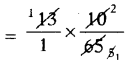= $$\frac{2}{1}$$
∴ 13 ÷ 6.5 = 2

(viii) 10.01 ÷ 11
10.01 ÷ 11
= $$\frac{1001}{100} \div \frac{11}{1}$$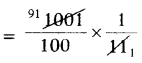= $$\frac{91}{100}$$
∴ 10.01 ÷ 11 = 0.91

(ix) 8 ÷ 0.32
8 ÷ 0.32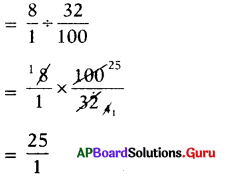∴ 8 ÷ 0.32 = 25(x) 320.1 ÷ 33
320.1 ÷ 33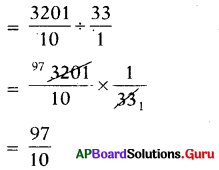∴ 320.1 ÷ 33 = 9.7

Question 3.
Solve the following divisions,
(i) 78.24 ÷ 0.2
78.24 ÷ 0.2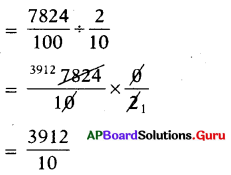∴ 78.24 ÷ 0.2 = 391.2

(ii) 4.845 ÷ 1.5
4.845 ÷ 1.5
= $$\frac{4845}{1000} \div \frac{15}{10}$$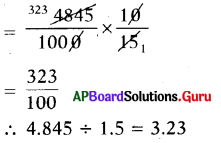(iii) 0.246 ÷ 0.6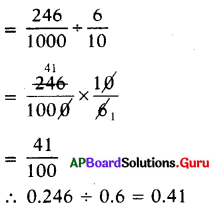(iv) 563.2 ÷ 2.2
563.2 ÷ 2.2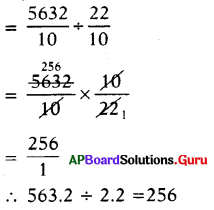∴ 563.2 ÷ 2.2 = 256

(v) 0.026 ÷ 0.13
0.026 ÷ 0.13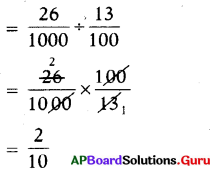∴ 0.026 ÷ 0.13 = 0.2

(vi) 4.347 ÷ 0.09
4.347 ÷ 0.09
$$\frac{4347}{1000} \div \frac{9}{100}$$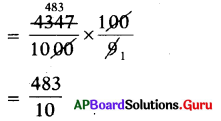∴ 4.347 ÷ 0.09 = 48.3

(vii) 3.9 ÷ 0.13
3.9 ÷ 0.13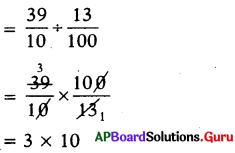∴ 3.9 ÷ 0.13 = 30

(viii) 2032 ÷ 0.8
20.32 ÷ 0.8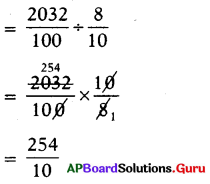∴ 20.32 ÷ 0.8 = 25.4

(ix) 24.4 ÷ 6.1
24.4 ÷ 6.1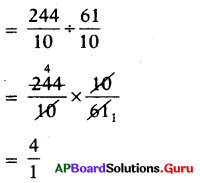∴ 24.4 ÷ 6.1 = 4(x) 2.164 ÷ 0.008
2.164 ÷ 0.008
$$\frac{2164}{1000} \div \frac{8}{1000}$$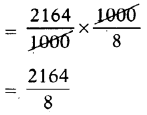∴ 2.164 ÷ 0.008 = 270.5

Question 4.
Solve the following.
(i) Divide 39.54 by 6
39.54 by 6
= 39.54 ÷ 6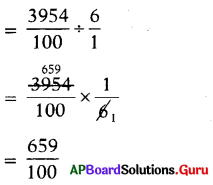∴ 39.54 ÷ 6 = 6.59

(ii) Divide 72 by 10
7.2 by 10
= 7.2 ÷ 10
= $$\frac{72}{10} \div \frac{10}{1}$$
= $$\frac{72}{10} \times \frac{1}{10}$$
= $$\frac{72 \times 1}{10 \times 10}=\frac{72}{100}$$
∴ 7.2 ÷ 10 = 0.72

(iii) Divide 5.2 by 1.3
5.2 by 1.3
= 5.2 ÷ 1.3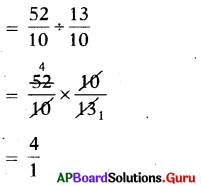∴ 5.2 ÷ 1.3 = 4

Question 5.
‘Sekhar travelled 154.5 km. in 5 hours with uniform speed on his bike. How much distance does he travel in one hour ?
Given, distance travelled in 5 hours = 154.5 km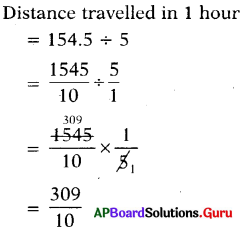∴ Distance travelled by Sekhar in 1 hour = 30.9 kmQuestion 6.
If a Mason worked 100 hours in 12.5 days to construct a wall, then how many hours he totally worked in a day?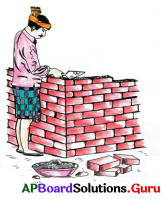Given number of hours worked in 12.5 days to.construct a wall = 100 hours.
Number of hours worked in one day
= 100 ÷ 12.5
= $$\frac{100}{1} \div \frac{125}{10}$$
= $$\frac{100}{1} \times \frac{10}{125}$$
= $$\frac{1000}{125}$$
∴ Number of hours worked by Mason in one day = 8 hours.

Question 7.
If the cost of dozen eggs is ₹ 61.80, then find the cost of an egg.
Given, cost of dozen eggs = ₹ 61.80
We know 1 dozen =12
Cost of one egg = 61.80 ÷ 12= $$\frac{515}{100}$$
∴ Cost of one egg = ₹ 5.15

Question 8.
If the price of a tablet strip containing 10 tablets is ₹ 26.5, then find the price of each tablet.
= $$\frac{265}{10} \div \frac{10}{1}$$
= $$\frac{265}{10} \times \frac{1}{10}$$
= $$\frac{265}{100}$$